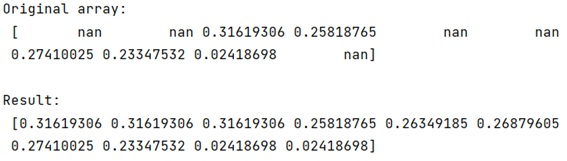# Replace NaN's with closest non-NaN value in NumPy Array

In this tutorial, we will learn how to replace NaN values with the closest non-NaN values in NumPy array? By Pranit Sharma Last updated : May 12, 2023

Suppose that we are given a NumPy array that contains some NaN values and we need to replace these NaN values with the closest numerical value (non-NaN values).

## How to replace NaN's in NumPy array with closest non-NaN value?

To replace NaN's in NumPy array with closest non-NaN value, you need to create/define a mask for checking NaN values and filter all the indices of NaN values using the defined mask. And then, linearly interpolate these values, and it will fill the nearest numerical (non-NaN) value. The following code snippets will do the same:

```mask = np.isnan(arr)
```

Let us understand with the help of an example,

## Python program to replace NaN's with closest non-NaN value in NumPy array

```# Import numpy
import numpy as np

# Creating an array
arr = np.array([
np.nan,
np.nan,
0.31619306,
0.25818765,
np.nan,
np.nan,
0.27410025,
0.23347532,
0.02418698,
np.nan])

# Display original array
print("Original array:\n",arr,"\n")

# Filling nan with closest numerical values

# Display result
print("Result:\n",arr)
```

### Output

```Original array:
[       nan        nan 0.31619306 0.25818765        nan        nan
0.27410025 0.23347532 0.02418698        nan]

Result:
[0.31619306 0.31619306 0.31619306 0.25818765 0.26349185 0.26879605
0.27410025 0.23347532 0.02418698 0.02418698]
```

### Output (Screenshot)